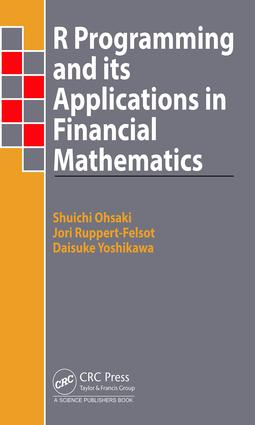R Programming and Its Applications in Financial Mathematics

1st Edition

CRC Press

248 pages | 4 Color Illus. | 62 B/W Illus.

Purchasing Options:\$ = USD
Hardback: 9781498766098
pub: 2018-02-12
SAVE ~\$31.00
\$155.00
\$124.00
x
eBook (VitalSource) : 9781315153810
pub: 2018-01-31
from \$28.98

FREE Standard Shipping!

Description

This book provides an introduction to R programming and a summary of financial mathematics.

It is not always easy for graduate students to grasp an overview of the theory of finance in an abstract form. For newcomers to the finance industry, it is not always obvious how to apply the abstract theory to the real financial data they encounter. Introducing finance theory alongside numerical applications makes it easier to grasp the subject.

Popular programming languages like C++, which are used in many financial applications are meant for general-purpose requirements. They are good for implementing large-scale distributed systems for simultaneously valuing many financial contracts, but they are not as suitable for small-scale ad-hoc analysis or exploration of financial data. The R programming language overcomes this problem. R can be used for numerical applications including statistical analysis, time series analysis, numerical methods for pricing financial contracts, etc.

This book provides an overview of financial mathematics with numerous examples numerically illustrated using the R programming language.

Preface

Introduction to R programming

Installation of R

Operators

Data structure

Functions

Control statements

Graphics

Reading and writing data

Packages

SECTION I: STATISTICS IN FINANCE

Statistical Analysis with R

Basic Statistics

Probability distribution and random numbers

Hypothesis testing

Regression Analysis

Yield curve analysis using principal component analysis?

Time Series Analysis with R

Preparation of time series data

Before applying for models

The application for AR model

Models extended from AR

Application of the time series analysis to finance: Pair trading

Nonlinear statistics with R

ARCH and GARCH

Nonparametric

Functional Data Analysis

SECTION II: BASIC THEORY OF FINANCE

Modern Portfolio Theory and CAPM

Mean-variance portfolio

Market portfolio

Derivation of CAPM

The extension of CAPM: Multi-factor model

The form of efficient frontier

Interest Rate Swap and Discount Factor

Interest rate swap

Pricing of interest rate swap and derivation of discount factors

Valuation of interest rate swap and risk analysis

Discrete Time Model: Tree Model

Single period binomial model

Multi period binomial model

Trinomial model

Continuous time model and the Black-Scholes Formula

Continuous rate of return

Itˆo’s lemma

The Black-Scholes formula

Implied volatility

SECTION III: NUMERICAL METHODS IN FINANCE

Monte Carlo Simulation

The basic concept of Monte Carlo simulation

Variance reduction method

Exotic option

Multi asset options

Control variates method

Derivative Pricing with Partial Differential Equation

Explicit method

Implicit method

Noise reduction via Kalman Filter

Introduction to Kalman filter

Nonlinear Kalman filter

SECTION IV: APPENDIX 237

A Optimization with R

A.1 Multi variate optimization problem

A.2 Efficient frontier by optimization problem

B Noise reduction via Kalman Filter

B.1 Introduction to Kalman filter

B.2 Nonlinear Kalman filter

C The other references on R

C.1 Information sources on R

C.2 R package on finance

References

Index

After finishing a Ph.D course at Kyoto University, Dr. Daisuke Yoshikawa worked for Mizuho-DL financial technology and Bank of Japan. Meanwhile, Dr. Yoshikawa published a few refereed journal papers on finance. Currently, Dr. Yoshikawa is working for Hokkai-Gakuen University as a lecturer.